﻿ 两点边值微分方程组多重正解的存在性 Existence of Multiple Positive Solutions of Differential Equation System with Two-Point Boundary Value

Advances in Applied Mathematics
Vol. 08  No. 02 ( 2019 ), Article ID: 28859 , 8 pages
10.12677/AAM.2019.82026

Existence of Multiple Positive Solutions of Differential Equation System with Two-Point Boundary Value

Xuan Zhong

Department of Mathematics, Nanjing University of Aeronautics and Astronautics, Nanjing Jiangsu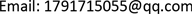Received: Jan. 23rd, 2019; accepted: Feb. 7th, 2019; published: Feb. 14th, 2019ABSTRACT

This paper deals with a class of two-point boundary value problems for differential equation system with parameters. Using fixed point index theory, existence of one positive solution and two positive solutions are obtained to this class of systems.

Keywords:Two-Point Boundary Value Problem, Fixed Point Index, First Eigenvalue, Green’s FunctionCopyright © 2019 by author and Hans Publishers Inc.1. 引言

$\left\{\begin{array}{l}{u}^{\left(4\right)}\left(t\right)=f\left(t,v\right),\text{ }\text{ }\text{\hspace{0.17em}}\text{\hspace{0.17em}}\text{ }\forall \left(t,v\right)\in \left[0,1\right]×\left[0,+\infty \right),\\ -{v}^{″}\left(t\right)=g\left(t,u\right),\text{ }\text{ }\text{ }\forall \left(t,u\right)\in \left[0,1\right]×\left[0,+\infty \right),\\ u\left(0\right)=u\left(1\right)={u}^{″}\left(0\right)={u}^{″}\left(1\right)=0,\\ v\left(0\right)=v\left(1\right)=0.\end{array}$

$\left\{\begin{array}{l}{u}^{\left(4\right)}\left(t\right)=\lambda {h}_{1}\left(t\right)f\left(t,u\left(t\right),v\left(t\right)\right),\text{ }\forall t\in \left[0,1\right],\\ -{v}^{″}\left(t\right)=\mu {h}_{2}\left(t\right)g\left(t,u\left(t\right),v\left(t\right)\right),\text{ }\forall t\in \left[0,1\right],\\ u\left(0\right)=u\left(1\right)={u}^{″}\left(0\right)={u}^{″}\left(1\right)=0,\\ v\left(0\right)=v\left(1\right)=0.\end{array}$ (1)

(H1) $f,g:\left[0,1\right]×\left[0,+\infty \right)×\left[0,+\infty \right)\to \left[0,+\infty \right)$ ，且 $f,g$ 连续；

(H2) ${h}_{i}\in {L}^{1}\left[0,1\right]$${h}_{i}\left(t\right)\ge 0$ ，对 $\forall t\in \left[0,1\right]$${\int }_{\frac{1}{4}}^{\frac{3}{4}}{h}_{i}\left(t\right)\text{d}t>0$ ，其中 $i=1,2$

(H3) $\lambda ,\mu >0$ ，且为常数。

$\begin{array}{l}{f}_{0}=\underset{\left(u,v\right)\to {0}^{+}}{\mathrm{lim}}\text{inf}\frac{f\left(u,v\right)}{u+{v}^{2}},{f}^{0}=\underset{\left(u，v\right)\to {0}^{+}}{\mathrm{lim}}\text{sup}\frac{f\left(u,v\right)}{u+{v}^{2}},{f}_{\infty }=\underset{\left(u,v\right)\to \infty }{\mathrm{lim}}\text{inf}\frac{f\left(u,v\right)}{u+\sqrt{v}},{f}^{\infty }=\underset{\left(u,v\right)\to \infty }{\mathrm{lim}}\text{sup}\frac{f\left(u,v\right)}{u+\sqrt{v}}\\ {g}_{0}=\underset{\left(u,v\right)\to {0}^{+}}{\mathrm{lim}}\text{inf}\frac{g\left(u,v\right)}{u+{v}^{2}},{g}^{0}=\underset{\left(u,v\right)\to {0}^{+}}{\mathrm{lim}}\text{sup}\frac{g\left(u,v\right)}{u+{v}^{2}},{g}_{\infty }=\underset{\left(u,v\right)\to \infty }{\mathrm{lim}}\text{inf}\frac{g\left(u,v\right)}{u+\sqrt{v}},{g}^{\infty }=\underset{\left(u,v\right)\to \infty }{\mathrm{lim}}\text{sup}\frac{g\left(u,v\right)}{u+\sqrt{v}}\end{array}$

$K=\left\{\left(u,v\right)\in E|u\left(t\right)\ge 0,v\left(t\right)\ge 0,\underset{t\in \left[\frac{1}{4},\frac{3}{4}\right]}{\mathrm{min}}u\left(\text{t}\right)\ge \frac{1}{4}‖u‖,\underset{t\in \left[\frac{1}{4},\frac{3}{4}\right]}{\mathrm{min}}v\left(\text{t}\right)\ge \frac{1}{4}‖v‖,\forall t\in \left[0,1\right]\right\}$

2. 预备知识

1) $‖Ax‖\le ‖x‖,\forall x\in P\cap \partial {\Omega }_{1};‖Ax‖\ge ‖x‖,\forall x\in P\cap \partial {\Omega }_{2}$

2) $‖Ax‖\le ‖x‖,\forall x\in P\cap \partial {\Omega }_{2};‖Ax‖\ge ‖x‖,\forall x\in P\cap \partial {\Omega }_{1}$

1、当 $t,s\in \left[0,1\right]$ 时，有 $0\le G\left(t,s\right)\le G\left(t,t\right)$$0\le G\left(t,s\right)\le G\left(s,s\right)$

2、 $\frac{1}{4}G\left(x,s\right)\le G\left(t,s\right),\text{\hspace{0.17em}}\forall t\in \left[\frac{1}{4},\frac{3}{4}\right],\text{\hspace{0.17em}}\forall x,s\in \left[0,1\right]$

$\left\{\begin{array}{l}u\left(t\right)=\lambda {\int }_{0}^{1}{\int }_{0}^{1}G\left(t,s\right)G\left(\tau ,s\right){h}_{1}\left(\tau \right)f\left(u\left(\tau \right),v\left(\tau \right)\right)\text{d}\tau \text{d}s,\text{ }\text{\hspace{0.17em}}\text{\hspace{0.17em}}\text{\hspace{0.17em}}\forall t\in \left[0,1\right]\\ v\left(t\right)=\mu {\int }_{0}^{1}G\left(t,s\right){h}_{2}\left(s\right)g\left(u\left(s\right),v\left(s\right)\right)\text{d}s,\text{ }\text{ }\text{ }\text{ }\text{ }\text{\hspace{0.17em}}\text{\hspace{0.17em}}\text{\hspace{0.17em}}\text{\hspace{0.17em}}\forall t\in \left[0,1\right]\text{ }\end{array}$

$\Phi \left(u,v\right)=\lambda {\int }_{0}^{1}{\int }_{0}^{1}G\left(t,s\right)G\left(\tau ,s\right){h}_{1}\left(\tau \right)f\left(u\left(\tau \right),v\left(\tau \right)\right)\text{d}\tau \text{d}s,\text{ }\text{\hspace{0.17em}}\text{\hspace{0.17em}}\forall t\in \left[0,1\right]$

$\Psi \left(u,v\right)=\mu {\int }_{0}^{1}G\left(t,s\right){h}_{2}\left(s\right)g\left(u\left(s\right),v\left(s\right)\right)\text{d}s,\text{ }\text{\hspace{0.17em}}\text{\hspace{0.17em}}\text{\hspace{0.17em}}\text{\hspace{0.17em}}\forall t\in \left[0,1\right]$

${P}_{1}u\left(t\right)={\int }_{0}^{1}{\int }_{0}^{1}G\left(t,s\right)G\left(\tau ,s\right){h}_{1}\left(\tau \right)u\left(\tau \right)\text{d}\tau \text{d}s,\text{\hspace{0.17em}}\text{\hspace{0.17em}}\text{ }\text{ }\text{ }\text{ }\text{ }\text{ }\text{\hspace{0.17em}}\forall u\in C\left[0,1\right]$

${P}_{2}v\left(t\right)={\int }_{0}^{1}G\left(t,s\right){h}_{2}\left(s\right)v\left(s\right)\text{d}s,\text{\hspace{0.17em}}\text{\hspace{0.17em}}\text{\hspace{0.17em}}\forall v\in C\left[0,1\right]$ $P\left(u,v\right)\left(t\right)=\left({P}_{1}u\left(t\right),{P}_{2}v\left(t\right)\right),\text{ }\text{ }\text{ }\text{ }\text{ }\text{ }\text{ }\text{ }\text{ }\text{ }\text{ }\text{ }\text{ }\text{ }\text{ }\forall \left(u,v\right)\in E$

$H\left(u,v\right)\left(t\right)=\left(\lambda f\left(u\left(t\right),v\left(t\right)\right),\mu g\left(u\left(t\right),v\left(t\right)\right)\right),\text{ }\text{ }\text{ }\text{ }\text{ }\text{ }\forall \left(u,v\right)\in K$

$\begin{array}{l}T\left(u,v\right)=PH\left(u,v\right)=\left(\lambda {P}_{1}u\left(t\right),\mu {P}_{2}u\left(t\right)\right)\\ =\left(\lambda {\int }_{0}^{1}{\int }_{0}^{1}G\left(t,s\right)G\left(\tau ,s\right){h}_{1}\left(\tau \right)f\left(u\left(\tau \right),v\left(\tau \right)\right)\text{d}\tau \text{d}s,\mu {\int }_{0}^{1}G\left(t,s\right){h}_{2}\left(s\right)g\left(u\left(s\right),v\left(s\right)\right)\text{d}s\right)\end{array}$

$\begin{array}{c}T\left(u,v\right)\left(t\right)=\left(\lambda {\int }_{0}^{1}{\int }_{0}^{1}G\left(t,s\right)G\left(\tau ,s\right){h}_{1}\left(\tau \right)f\left(u\left(\tau \right),v\left(\tau \right)\right)\text{d}\tau \text{d}s,\mu {\int }_{0}^{1}G\left(t,s\right){h}_{2}\left(s\right)g\left(u\left(s\right),v\left(s\right)\right)\text{d}s\right)\\ \ge \frac{1}{4}\left(\lambda {\int }_{0}^{1}{\int }_{0}^{1}G\left(x,s\right)G\left(\tau ,s\right){h}_{1}\left(\tau \right)f\left(u\left(\tau \right),v\left(\tau \right)\right)\text{d}\tau \text{d}s,\mu {\int }_{0}^{1}G\left(x,s\right){h}_{2}\left(s\right)g\left(u\left(s\right),v\left(s\right)\right)\text{d}s\right)\\ =\frac{1}{4}T\left(u,v\right)\left(x\right)\end{array}$

$\underset{t\in \left[\frac{1}{4},\frac{3}{4}\right]}{\mathrm{min}}\Phi \left(u,v\right)\left(t\right)\ge \frac{1}{4}‖\Phi \left(u,v\right)‖\text{ }$$\underset{t\in \left[\frac{1}{4},\frac{3}{4}\right]}{\mathrm{min}}\Psi \left(u,v\right)\left(t\right)\ge \frac{1}{4}‖\Psi \left(u,v\right)‖$ ，所以 $T\left(K\right)\subset K$

${\phi }_{i}\in C\left[0,1\right]$ ，满足 ${\phi }_{i}\left(t\right)\ge 0,\text{\hspace{0.17em}}\forall t\in \left[0,1\right],\text{\hspace{0.17em}}{\phi }_{i}\left({t}_{0}\right)>0$${\phi }_{i}\left(t\right)=0,\text{\hspace{0.17em}}t\notin \left[\alpha ,\beta \right]$ ，则

$\begin{array}{c}{P}_{1}{\phi }_{1}\left(t\right)={\int }_{0}^{1}{\int }_{0}^{1}G\left(t,s\right)G\left(\tau ,s\right){h}_{1}\left(\tau \right){\phi }_{1}\left(\tau \right)\text{d}\tau \text{d}s\\ \ge {\int }_{\alpha }^{\beta }{\int }_{0}^{1}G\left(t,s\right)G\left(\tau ,s\right){h}_{1}\left(\tau \right){\phi }_{1}\left(\tau \right)\text{d}\tau \text{d}s>0,\text{ }\forall t\in \left[\alpha ,\beta \right]\end{array}$

${P}_{2}{\phi }_{2}\left(t\right)={\int }_{0}^{1}G\left(t,s\right){h}_{2}\left(s\right){\phi }_{2}\left(s\right)\text{d}s>{\int }_{\alpha }^{\beta }G\left(t,s\right){h}_{2}\left(s\right){\phi }_{2}\left(s\right)\text{d}s>0,\text{ }\text{ }\text{ }\text{ }\text{ }\text{ }\text{ }\text{ }\text{ }\text{ }\text{ }\text{ }\text{ }\forall t\in \left[\alpha ,\beta \right]$

${\lambda }_{1}$ 为线性边值问题 $\left\{\begin{array}{l}{u}^{\left(4\right)}\left(t\right)=\lambda {h}_{1}\left(t\right)u\left(t\right),\text{ }\forall t\in \left(0,1\right)\\ u\left(0\right)=u\left(1\right)={u}^{″}\left(0\right)={u}^{″}\left(1\right)=0\end{array}$ 的第一个特征值， ${\mu }_{1}$ 为线性边值问题 $\left\{\begin{array}{l}-{v}^{″}\left(t\right)=\mu {h}_{2}\left(t\right)v\left(t\right),\text{\hspace{0.17em}}\text{\hspace{0.17em}}\forall t\in \left(0,1\right)\\ v\left(0\right)=v\left(1\right)=0\end{array}$ 的第一个特征值，则 ${\lambda }_{1},{\mu }_{1}>0$ 。从而 ${P}_{1}$ 的谱半径 ${r}_{1}=\frac{1}{{\lambda }_{1}}>0$${P}_{2}$ 的谱半径 ${r}_{2}=\frac{1}{{\mu }_{1}}>0$ 。假设它们相对应的特征函数分别为 ${\phi }_{1},{\phi }_{2}$ 。则满足 ${\int }_{0}^{1}{h}_{i}\left(t\right){\phi }_{i}\left(t\right)>0$ ，否则如果 ${\int }_{0}^{1}{h}_{i}\left(t\right){\phi }_{i}\left(t\right)=0$ ，则

${r}_{1}{\phi }_{1}\left(t\right)={P}_{1}{\phi }_{1}\left(t\right)={\int }_{0}^{1}{\int }_{0}^{1}G\left(t,s\right)G\left(\tau ,s\right){h}_{1}\left(\tau \right){\phi }_{1}\left(\tau \right)\text{d}\tau \text{d}s\le {M}^{2}{\int }_{0}^{1}{h}_{1}\left(\tau \right){\phi }_{1}\left(\tau \right)\text{d}\tau =0$

${r}_{2}{\phi }_{2}\left(t\right)={P}_{2}{\phi }_{2}\left(t\right)={\int }_{0}^{1}G\left(t,s\right){h}_{2}\left(s\right){\phi }_{2}\left(s\right)\text{d}s

$z\left(u,v\right)={\int }_{0}^{1}\left[{\phi }_{1}\left(t\right){h}_{1}\left(t\right)u\left(t\right)+{\phi }_{2}\left(t\right){h}_{2}\left(t\right)v\left(t\right)\right]\text{d}t,\text{ }\forall \left(u,v\right)\in E$

$z\left(u,v\right)\ge \frac{1}{4}\left(‖u‖{m}_{1}+‖v‖{m}_{2}\right)\ge m‖\left(u,v\right)‖$ (2)

$\begin{array}{c}z\left(P\left(u,v\right)\right)={\int }_{0}^{1}\left[{\phi }_{1}\left(t\right){h}_{1}\left(t\right){\int }_{0}^{1}{\int }_{0}^{1}G\left(t,s\right)G\left(\tau ,s\right){h}_{1}\left(\tau \right)u\left(\tau \right)\text{d}\tau \text{d}s+{\phi }_{2}\left(t\right){h}_{2}\left(t\right){\int }_{0}^{1}G\left(t,s\right){h}_{2}\left(s\right)v\left(s\right)\text{d}s\right]\text{d}t\\ ={\int }_{0}^{1}\left[{\phi }_{1}\left(t\right){h}_{1}\left(t\right){\int }_{0}^{1}{\int }_{0}^{1}G\left(t,s\right)G\left(\tau ,s\right){h}_{1}\left(\tau \right)u\left(\tau \right)\text{d}\tau \text{d}s+{\phi }_{2}\left(t\right){h}_{2}\left(t\right){\int }_{0}^{1}G\left(t,\tau \right){h}_{2}\left(\tau \right)v\left(\tau \right)\text{d}\tau \right]\text{d}t\\ ={\int }_{0}^{1}\left[{h}_{1}\left(\tau \right)u\left(\tau \right){\int }_{0}^{1}{\int }_{0}^{1}G\left(\tau ,s\right)G\left(s,t\right){h}_{1}\left(t\right){\phi }_{1}\left(t\right)\text{d}t\text{d}s+{h}_{2}\left(\tau \right)v\left(\tau \right){\int }_{0}^{1}G\left(\tau ,t\right){h}_{2}\left(t\right){\phi }_{2}\left(t\right)\text{d}t\right]\text{d}\tau \end{array}$

$z\left(P\left(u,v\right)\right)={\int }_{0}^{1}\left[{h}_{1}\left(\tau \right)u\left(\tau \right){r}_{1}{\phi }_{1}\left(\tau \right)+{h}_{2}\left(\tau \right)v\left(\tau \right){r}_{2}{\phi }_{2}\left(\tau \right)\right]\text{d}\tau$ (3)

3. 主要结果

1) 由 $\lambda {f}^{0}<{\lambda }_{1},\mu {g}^{0}<{\mu }_{1}$ 可得 $\exists \delta \in \left(0,1\right),d>0$ 且充分小，使得当 $\left(u,v\right)\in K,‖\left(u,v\right)‖\le d$ 时，对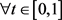，恒有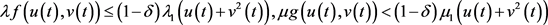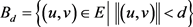，假设存在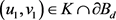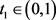满足，则由(3)式得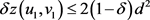，由(2)式得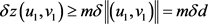，即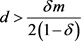，这与d充分小矛盾。根据不动点指数的同伦不变性得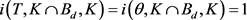2) 由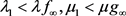可得，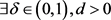，使得当时，有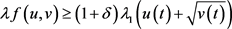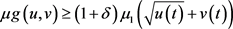。又当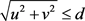时，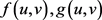有界，故存在常数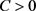，使得当时，对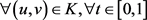，恒有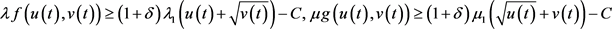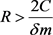，令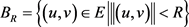，假设存在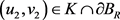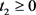满足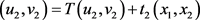，其中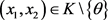，则由(3)可得1) 由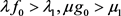可得，使得当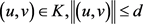时，对，有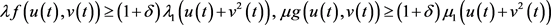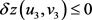，这与(2)式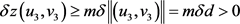矛盾，从而假设不成立。由不动点指数的缺方向性知，当d充分小时，有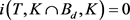2) 又由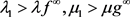可得，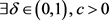，使得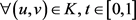时，有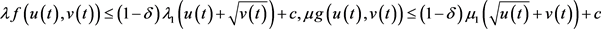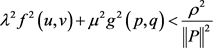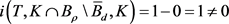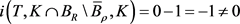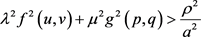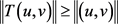，故由锥压缩定理知，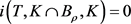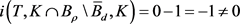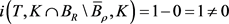Existence of Multiple Positive Solutions of Differential Equation System with Two-Point Boundary Value[J]. 应用数学进展, 2019, 08(02): 227-234. https://doi.org/10.12677/AAM.2019.82026

1. 1. 郭大钧. 非线性泛函分析[M]. 第3版. 济南: 山东科技出版社, 1985: 243-244.

2. 2. 姚庆六, 白占兵. 的边值问题的正解存在性[J]. 数学年刊: 中文版, 1999(5): 575-578.

3. 3. 席莉静. 一类算子方程的多重正解及对奇异边值问题的应用[J]. 苏州科技大学学报(自然科学版), 2004, 21(3): 26-30.

4. 4. 陈丽珍, 李刚. 四阶非线性微分方程组正解的存在性[J]. 扬州大学学报(自然科学版), 2008, 11(4): 25-28.

5. 5. 王全义, 邹黄辉. 一类四阶奇异非线性积分边值问题正解的存在性[J]. 华侨大学学报, 2014, 35(1): 83-87.

6. 6. 赵微. 一类四阶微分方程m点边值问题的正解[J]. 四川师范大学学报(自然科学版), 2017, 40(6): 791-796.

7. 7. Jankowski, T. (2010) Positive Solutions for Fourth-Order Differential Equations with Deviating Argu-ments and Integral Boundary Conditions. Nonlinear Analysis, 73, 1289-1299. https://doi.org/10.1016/j.na.2010.04.055

8. 8. 马田田, 赵增勤. 非线性微分方程组边值问题多个正解的存在性[J]. 工程数学学报, 2009, 26(6): 1050-1056.

9. 9. 周韶林, 吴红萍, 韩晓玲. 一类四阶三点边值问题正解的存在性[J]. 四川大学学报(自然科学版), 2014, 51(1): 11-15.